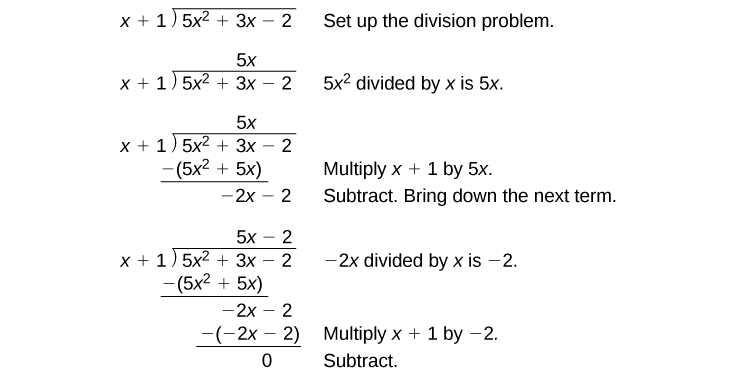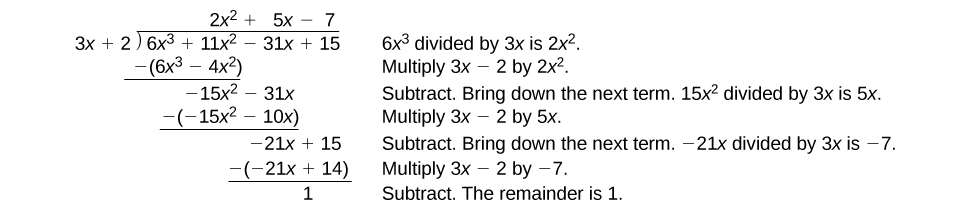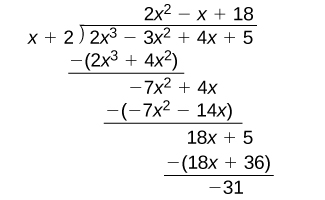# 5.4 Dividing polynomials  (Page 2/6)

 Page 2 / 6

Given a polynomial and a binomial, use long division to divide the polynomial by the binomial.

1. Set up the division problem.
2. Determine the first term of the quotient by dividing the leading term of the dividend by the leading term of the divisor.
3. Multiply the answer by the divisor and write it below the like terms of the dividend.
4. Subtract the bottom binomial    from the top binomial.
5. Bring down the next term of the dividend.
6. Repeat steps 2–5 until reaching the last term of the dividend.
7. If the remainder is non-zero, express as a fraction using the divisor as the denominator.

## Using long division to divide a second-degree polynomial

Divide $\text{\hspace{0.17em}}5{x}^{2}+3x-2\text{\hspace{0.17em}}$ by $\text{\hspace{0.17em}}x+1.$The quotient is $\text{\hspace{0.17em}}5x-2.\text{\hspace{0.17em}}$ The remainder is 0. We write the result as

$\frac{5{x}^{2}+3x-2}{x+1}=5x-2$

or

$5{x}^{2}+3x-2=\left(x+1\right)\left(5x-2\right)$

## Using long division to divide a third-degree polynomial

Divide $\text{\hspace{0.17em}}6{x}^{3}+11{x}^{2}-31x+15\text{\hspace{0.17em}}$ by $\text{\hspace{0.17em}}3x-2.\text{\hspace{0.17em}}$There is a remainder of 1. We can express the result as:

$\frac{6{x}^{3}+11{x}^{2}-31x+15}{3x-2}=2{x}^{2}+5x-7+\frac{1}{3x-2}$

Divide $\text{\hspace{0.17em}}16{x}^{3}-12{x}^{2}+20x-3\text{\hspace{0.17em}}$ by $\text{\hspace{0.17em}}4x+5.\text{\hspace{0.17em}}$

$4{x}^{2}-8x+15-\frac{78}{4x+5}$

## Using synthetic division to divide polynomials

As we’ve seen, long division of polynomials can involve many steps and be quite cumbersome. Synthetic division is a shorthand method of dividing polynomials for the special case of dividing by a linear factor whose leading coefficient is 1.

To illustrate the process, recall the example at the beginning of the section.

Divide $\text{\hspace{0.17em}}2{x}^{3}-3{x}^{2}+4x+5\text{\hspace{0.17em}}$ by $\text{\hspace{0.17em}}x+2\text{\hspace{0.17em}}$ using the long division algorithm.

The final form of the process looked like this:There is a lot of repetition in the table. If we don’t write the variables but, instead, line up their coefficients in columns under the division sign and also eliminate the partial products, we already have a simpler version of the entire problem.Synthetic division carries this simplification even a few more steps. Collapse the table by moving each of the rows up to fill any vacant spots. Also, instead of dividing by 2, as we would in division of whole numbers, then multiplying and subtracting the middle product, we change the sign of the “divisor” to –2, multiply and add. The process starts by bringing down the leading coefficient.We then multiply it by the “divisor” and add, repeating this process column by column, until there are no entries left. The bottom row represents the coefficients of the quotient; the last entry of the bottom row is the remainder. In this case, the quotient is $\text{\hspace{0.17em}}2{x}^{2}–7x+18\text{\hspace{0.17em}}$ and the remainder is $\text{\hspace{0.17em}}–31.\text{\hspace{0.17em}}$ The process will be made more clear in [link] .

## Synthetic division

Synthetic division is a shortcut that can be used when the divisor is a binomial in the form $\text{\hspace{0.17em}}x-k\text{\hspace{0.17em}}$ where $\text{\hspace{0.17em}}k\text{\hspace{0.17em}}$ is a real number. In synthetic division    , only the coefficients are used in the division process.

Given two polynomials, use synthetic division to divide.

1. Write $\text{\hspace{0.17em}}k\text{\hspace{0.17em}}$ for the divisor.
2. Write the coefficients of the dividend.
3. Bring the lead coefficient down.
4. Multiply the lead coefficient by $\text{\hspace{0.17em}}k.\text{\hspace{0.17em}}$ Write the product in the next column.
5. Add the terms of the second column.
6. Multiply the result by $\text{\hspace{0.17em}}k.\text{\hspace{0.17em}}$ Write the product in the next column.
7. Repeat steps 5 and 6 for the remaining columns.
8. Use the bottom numbers to write the quotient. The number in the last column is the remainder and has degree 0, the next number from the right has degree 1, the next number from the right has degree 2, and so on.

how do I set up the problem?
what is a solution set?
Harshika
find the subring of gaussian integers?
Rofiqul
hello, I am happy to help!
Abdullahi
hi mam
Mark
find the value of 2x=32
divide by 2 on each side of the equal sign to solve for x
corri
X=16
Michael
Want to review on complex number 1.What are complex number 2.How to solve complex number problems.
Beyan
yes i wantt to review
Mark
use the y -intercept and slope to sketch the graph of the equation y=6x
how do we prove the quadratic formular
Darius
hello, if you have a question about Algebra 2. I may be able to help. I am an Algebra 2 Teacher
thank you help me with how to prove the quadratic equation
Seidu
may God blessed u for that. Please I want u to help me in sets.
Opoku
what is math number
4
Trista
x-2y+3z=-3 2x-y+z=7 -x+3y-z=6
can you teacch how to solve that🙏
Mark
Solve for the first variable in one of the equations, then substitute the result into the other equation. Point For: (6111,4111,−411)(6111,4111,-411) Equation Form: x=6111,y=4111,z=−411x=6111,y=4111,z=-411
Brenna
(61/11,41/11,−4/11)
Brenna
x=61/11 y=41/11 z=−4/11 x=61/11 y=41/11 z=-4/11
Brenna
Need help solving this problem (2/7)^-2
x+2y-z=7
Sidiki
what is the coefficient of -4×
-1
Shedrak
the operation * is x * y =x + y/ 1+(x × y) show if the operation is commutative if x × y is not equal to -1
An investment account was opened with an initial deposit of \$9,600 and earns 7.4% interest, compounded continuously. How much will the account be worth after 15 years?
lim x to infinity e^1-e^-1/log(1+x)
given eccentricity and a point find the equiationByByBy Brooke DelaneyBy Richley CrapoBy Szilárd JankóBy OpenStaxBy Jazzycazz JacksonBy OpenStaxBy Stephen VoronBy Brooke DelaneyBy OpenStaxBy Frank Levy